### Why should I choose AnalystNotes?

AnalystNotes specializes in helping candidates pass. Period.

##### Subject 1. Cash Flow Projections
The incremental cash flows from a typical project can be classified into three categories:

• initial investment outlay. It includes the up-front cost of fixed assets associated with the project plus any increase in net working capital.

• operating cash flows over the project's life. These are the incremental cash inflows over the project's economic life. Annual operating cash flows equal after-tax operating income plus depreciation.

• terminal year cash flows. They include the after-tax salvage value of the fixed assets (adjusted for taxes if assets are not sold at book value), and the recovery of the net working capital.

For each year of the project's economic life, the net cash flow is determined as the sum of the cash flows from each of the three categories. These annual net cash flows, along with the project's cost of capital, are then plotted on a time line and used to calculate the project's NPV and IRR.

An expansion project is one where a firm invests in new assets to increase sales. Replacement analysis involves the decision of whether or not to replace an existing asset with a new asset: the cash flows from the old asset must be considered in replacement decisions. Specifically, in a replacement project, the cash flows from selling old assets should be used to offset the initial investment outlay. That is, the cash flows relevant to an investing decision are the incremental cash flows: the cash flows the company realizes with the investment compared to the cash flows the company would realize without the investment. You also need to compare the revenue/cost/depreciation before and after the replacement to identify changes in these elements.

There are four steps to be followed to make capital budgeting decisions (both for expansion and replacement):

• Estimate the initial investment outlay.
• Estimate the annual after-tax operating cash flows. Notice that depreciation schedules affect taxable income, taxes paid, and annual after-tax cash flows.
• Estimate the terminal year after-tax cash flow.
• Make the decision, using one or more of the following methods: IRR (or Modified IRR), NPV, Payback (or Discounted Payback).

Expansion Project Example

XYZ Inc. is considering a project to build a new plant.

• The plant would begin operations on 01/01/2005, and the first operating cash flows would occur on 12/31/2005 (the company's policy is to assume that operating cash flows occur at the end of each year).
• The marginal federal-plus-state tax rate is 40%.
• The cost of capital (WACC) is 12%. The project is assumed to have the same risk as an average project so the 12% should be used as the hurdle rate.
• The project's estimated economic life is 4 years.
• Annual sales brought by the new plant are estimated at \$40 million.
• Variable manufacturing costs would total 60% of sales.
• Fixed overhead costs (excluding depreciation) would be \$5 million a year.

Investments (all initial investments would occur on 12/31/2004):

• A building would be bought at a cost of \$12 million. For depreciation purposes the building would fall into the MACRS 39-year class. The building would have a market value of \$7.5 million and a book value of \$10.908 million at the end of the project.
• The necessary equipment would be purchased at a cost of \$8 million. It would fall into the MACRS 5-year class. It would have a market value of \$2 million and a book value of \$1.36 million at the end of the project.
• Net working capital: \$6 million. It will be fully recovered at the end of the project.

Relevant MACRS depreciation rates are as follows:Step 1. Estimate the initial investment outlay.

Initial investment outlay = Price of building + Price of equipment + ΔNWC = 12 + 8 + 6 = \$26 million.

Step 2. Estimate the operating cash flows:

Operating Cash Flow = (Revenue - Cost) (1 - t) + (Depreciation) (t)

• Total cost = variable costs + fixed costs = 40 x 60% + 5 = \$29 million.
• Yearly Depreciation Expense = Depreciable Basis x Recovery Percentage under MACRS

CF1 = (40 - 29) x (1 - 0.4) + [12 x 0.013 + 8 x 0.2] x 0.4 = \$7.302 million.
CF2 = (40 - 29) x (1 - 0.4) + [12 x 0.026 + 8 x 0.32] x 0.4 = \$7.749 million.
CF3 = (40 - 29) x (1 - 0.4) + [12 x 0.026 + 8 x 0.19] x 0.4 = \$7.333 million.
CF4 = (40 - 29) x (1 - 0.4) + [12 x 0.026 + 8 x 0.12] x 0.4 = \$7.109 million.

Step 3. Estimate the terminal year cash flows:

Let's first compute the after-tax salvage values of building and equipment:

After-Tax Salvage Value = Before-Tax Salvage Value - Tax = Before-Tax Salvage Value - [Before-Tax Salvage Value - End Book Value] x Tax Rate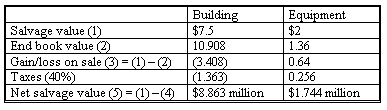Terminal Year Cash Flow = After-Tax Salvage Values of Fixed Assets + ΔNWC = 8.863 + 1.744 + 6 = \$16.667 million.

Step 4. Making the decision:

Now we have a cash flow time line of the project (millions of dollars):Given the cost of capital of 12%, we can calculate the NPV as follows: NPV = -26 + 7.302/(1.12)1 + 7.749/(1.12)2 + 7.333/(1.12)3 + 23.716/(1.12)4 = \$6.989 million > 0.

Decision: since NPV > 0, XYZ Inc. should accept the project.

If we use the IRR method:

NPV = -26 + 7.302/(1 + IRR)1 + 7.749/(1 + IRR)2 + 7.333/(1 + IRR)3 + 23.716/(1 + IRR)4 = 0 ==> IRR = 21.9%, which is greater than WACC.

Replacement Project Example

XYZ Inc. is considering a project to replace an existing machine.

• The machine would begin operations on 01/01/2005, and the first operating cash flows would occur on 12/31/2005 (again, the company's policy is to assume that operating cash flows occur at the end of each year).
• The marginal federal-plus-state tax rate is 40%.
• The cost of capital (WACC) is 12%. The project's cost of capital, however, is assumed to be 11.5%.
• The new machine's estimated economic life is 5 years. It can be purchased for \$12,000 on 12/31/2004. It falls into a MACRS 3-year class. At the end of the project the machine can be sold at \$2,000, while the book value will be \$0 since it will be fully depreciated.
• The new machines can cut annual operating costs from \$7,000 to \$4,000. This will raise before-tax profits by \$3,000.
• NWC requirements will increase by \$1,000 at the time of replacement. The \$1,000 will be fully recovered at the end of the project.
• If the new machine is acquired, the old one will be sold.
• The old machine was purchased 10 years ago at a cost of \$7,500. It had an expected life of 15 years at the time of purchase. The salvage value will be 0. It's being depreciated on a straight-line bases (that is, the annual depreciation expense is \$7,500/15 = \$500). Its market value on 12/31/2004 will be \$1,000, well below its book value of \$2,500.

Relevant MACRS depreciation rates are as follows: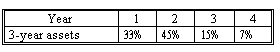Step 1. Estimate the initial investment outlay:

In a replacement project, the cash flows from selling the old machine should be used to offset the initial investment outlay. This is different from an expansion project where no old machine is involved.

Cash flows from selling the old machine is computed as below: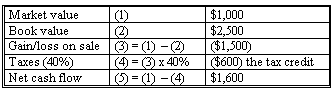Therefore, the initial investment outlay is computed as:

Initial investment outlay = Price of new machine - cash inflow from selling old machine + ΔNWC = 12,000 - 1,600 + 1,000 = \$11,400.

Step 2. Estimate the operating cash flows:

Operating Cash Flow = (Revenue - Cost) (1 - t) + (Depreciation) (t)

In a replacement project, we have to compare the revenue/cost/depreciation before and after the replacement to identify changes in these elements. This is not necessary in an expansion project.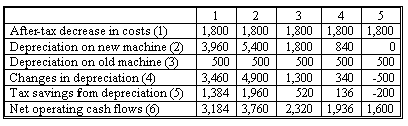(1): 0 - (4,000 - 7,000) x (1 - 0.4) = 1,800.
(2): calculated based on the 3-year class MACRS rates.
(4) = (2) - (3).
(5) = (4) x 0.4.
(6) = (1) + (5)

Step 3. Estimate the terminal year cash flows:

Terminal Year Cash Flow = After-tax Salvage Values of Fixed Assets + ΔNWC

For the new machine, the after-tax salvage value = before-tax salvage value - tax = before-tax salvage value - (before-tax salvage value - end book value) x tax rate = 2,000 - (2,000 - 0) x 0.4 = \$1,200.

Terminal Year Cash Flow = 1,200 + 1,000 = \$2,200.

Step 4. Making the decision:

Now we have a cash flow time line of the project: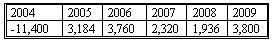Given the cost of capital of 11.5%, we can calculate the NPV as follows:

NPV = -11,400 + 3,184/(1.115)1 + 3,760/(1.115)2 + 2,320/(1.115)3 + 1,936/(1.115)4 + 3,800/(1.115)5 = -\$389 < 0.
Decision: since NPV < 0, XYZ Inc. should not accept the project.

If we use the IRR method, the IRR will be 10.1% which is less than the 11.5% hurdle rate. This will also leads to the decision not to accept the project.

The key to estimating the incremental cash flows for the replacement project is to compare the cash flows that occur with the new investment to the cash flows that would have occurred without the new investment.

Learning Outcome Statements

a. calculate the yearly cash flows of expansion and replacement capital projects and evaluate how the choice of depreciation method affects those cash flows;

CFA® 2023 Level I Curriculum, Volume 3, Module 19

User Comment
sarath Use only the NPV method. Also prepare a time based cash flow diagram. First calculate for the intial year, then hte net cash flows for teh all the years and then the terminating cash flow.
ryuz3 In the CFA book on pg 29, it says to add back depreciaton without first multiplying by the tax rate. Anyone know the reason for the discrepancy?
jmcneal All of depreciation is added back if you take taxes from revenue-cost-depreciation. IF you do (Revenue - cost)*(1-T) then you add back Depriacition after multiplying the tax rate. Just two different forms of the same equation.
BryonBUI Refer to CFAI text p31. Eqn 7 and 8 are equivalent
darbyland so does it mean if adjust the CF only and only if nominal interest rate is used to derive WACC?